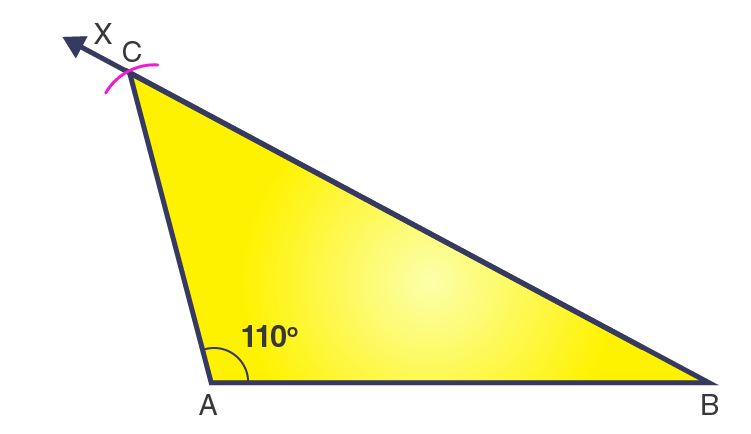# Construct an isosceles triangle in which the lengths of each of its equal sides is 6.5 cm and the angle between them is 110o.

SolutionThe steps for construction are given below:

1. Draw a line segment AB = 6.5 cm.

2. From point A, draw a ray AX to making an angle of 110o i.e. ∠XAB = 110o.

3. Along AX, set off AC = 6.5cm.

4. Join CB.

Then, ΔABC obtained is the required isosceles triangle.(0)(0)